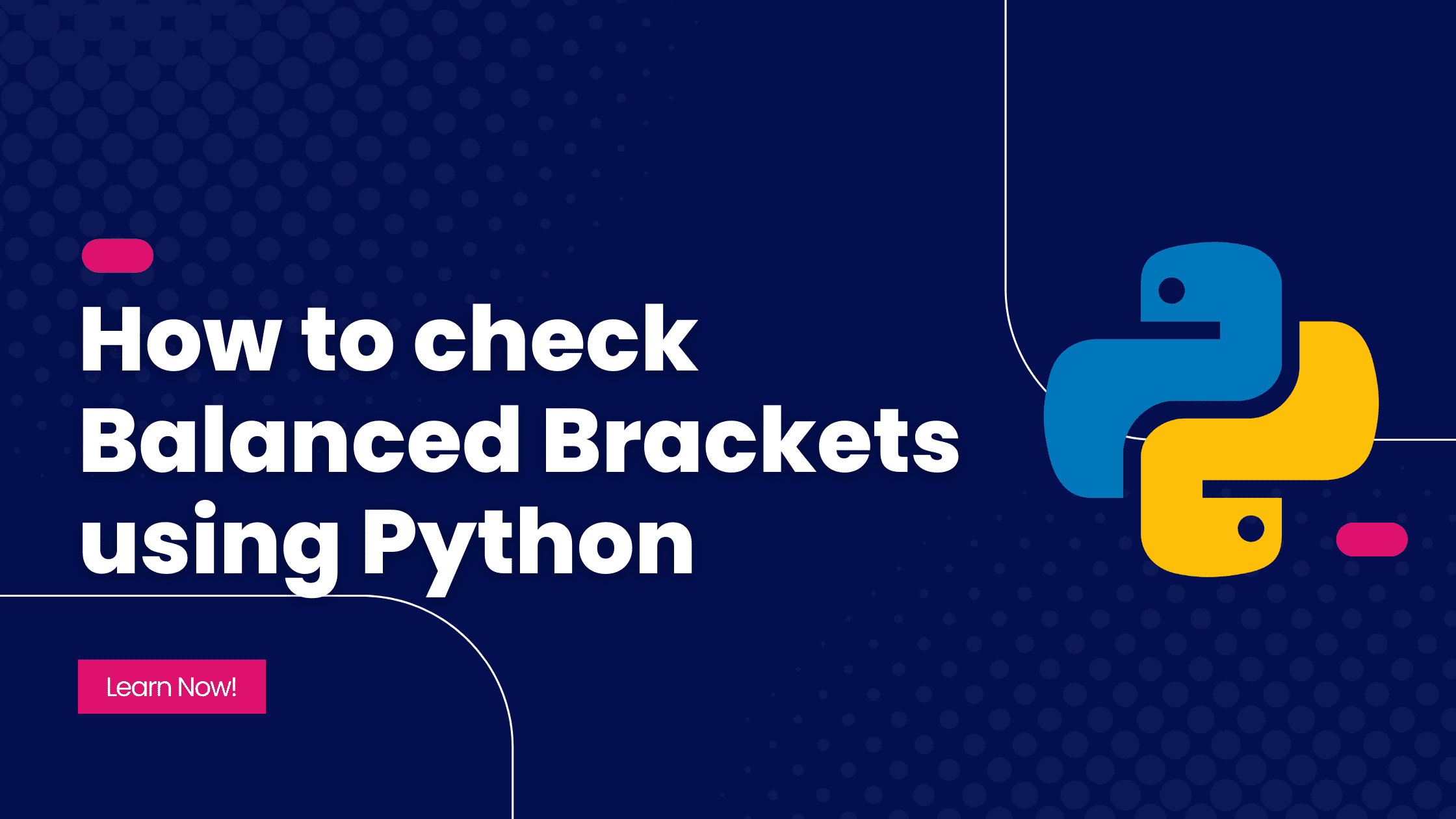# How to check Balanced Brackets using Python

Data Structures PYTHON SHORTS SERIES# Problem Statement

A bracket is considered to be any one of the following characters: `(``)``{``}``[`, or `]`.

Two brackets are considered to be a matched pair if an opening bracket (i.e., `(``[`, or `{`) occurs to the left of a closing bracket (i.e., `)``]`, or `}`of the exact same type. There are three types of matched pairs of brackets: `[]``{}`, and `()`.

A matching pair of brackets is not balanced if the set of brackets it encloses are not matched. For example, `{[(])}` is not balanced because the contents in between `{` and `}` are not balanced. The pair of square brackets enclose a single, unbalanced opening bracket, `(`, and the pair of parentheses encloses a single, unbalanced closing square bracket, `]`.

By this logic, we say a sequence of brackets is balanced if the following conditions are met:

• It contains no unmatched brackets.
• The subset of brackets enclosed within the confines of a matched pair of brackets is also a matched pair of brackets.

Given a string containing only brackets, determine whether the brackets are balanced. If the string is balanced, return `YES`. Otherwise, return `NO`.

# Algorithm

The problem can be solved using various methods, but we will be using a Stack here. Stack is a linear data structure that stores data in a Last In, First Out (LIFO) fashion, which means the element which is pushed last will be the first one to be popped out. If the closing bracket does not correspond to the opening bracket, then we stop and say that the brackets are not balanced. Whenever we get an opening bracket, we push it into the stack, while whenever we encounter a closing bracket, we pop the last inserted element from the stack. If the closing bracket doesn't pair with the opening bracket, we stop and return `NO` because the brackets are not balanced.

We also check if the stack is empty at the end. If it is not, the brackets are not balanced.

The time complexity for the algorithm is `O(n)` where n is the length of the bracket string. The space complexity will also be `O(n)` because we are using a stack of size `n`.

# Solution

Let us define a function to check if the brackets are balanced:

``````opening_brackets = ["(", "{", "["]
closing_brackets = [")", "}", "]"]

def is_balanced(bracket_string):
bracket_stack = []

for bracket in bracket_string:
if bracket in opening_brackets:
bracket_stack.append(bracket)

elif bracket in closing_brackets:
if len(bracket_stack) == 0:
return "NO"
popped_bracket = bracket_stack.pop()
if not brackets_correspond(popped_bracket, bracket):
return "NO"

if len(bracket_stack) != 0:
return "NO"

return "YES"

def brackets_correspond(opening, closing):
if opening == '(' and closing == ')':
return True
if opening == '{' and closing == '}':
return True
if opening == '[' and closing == ']':
return True
return False``````

First of all, we defined two lists - `opening_brackets` and `closing_brackets` containing opening and closing brackets respectively. Inside the `is_balanced()` function, we declare an empty list(or stack) called `bracket_stack`.

Now, we start iterating over the `bracket_string`. Whenever we find an opening bracket, we push it into the `bracket_stack`. However, if we encounter a closing bracket, we first check if the `bracket_stack` is empty. If it is, it means, we have an extra closing bracket, i.e., the brackets are not balanced. Hence, we return a `NO`. If the `bracket_stack` is not empty, we pop out the last inserted bracket and store it in a variable called `popped_bracket`. We then compare this `popped_bracket` with the current bracket and check if they correspond to each other. If they don't we return a `NO`. If they correspond to each other, we continue the iteration.

After the iteration completes, we check if the stack is empty or not. If not, we have extra brackets and hence the brackets are not balanced. So we return `NO`.  If everything goes fine, we return a `YES` at the end.

# Run the code

You can test the code from the above REPL.

# Conclusion

This was a basic use of Stack data structure. You can find this problem on various coding platforms.

I hope the algorithm and solution were clear to you. Thanks for reading!